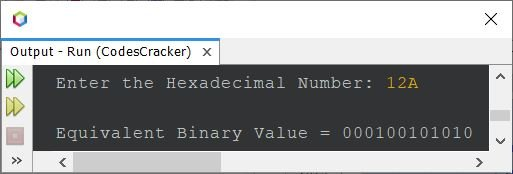# Java Program to Convert Hexadecimal to Binary

If you're not aware about, how the hexadecimal to binary conversion takes place, then refer to Hexadecimal to Binary Conversion. Now let's move on to the program.

## Hexadecimal to Binary Conversion in Java

The question is, write a Java program to convert hexadecimal number to binary. The hexadecimal number must be received by user at run-time. The program given below is its answer:

```import java.util.Scanner;

public class CodesCracker
{
public static void main(String[] args)
{
int i=0, len;

Scanner s = new Scanner(System.in);

System.out.print("\nEquivalent Binary Value = ");
while(i<len)
{
switch(hexDigit[i])
{
case '0':
System.out.print("0000");
break;
case '1':
System.out.print("0001");
break;
case '2':
System.out.print("0010");
break;
case '3':
System.out.print("0011");
break;
case '4':
System.out.print("0100");
break;
case '5':
System.out.print("0101");
break;
case '6':
System.out.print("0110");
break;
case '7':
System.out.print("0111");
break;
case '8':
System.out.print("1000");
break;
case '9':
System.out.print("1001");
break;
case 'a':
case 'A':
System.out.print("1010");
break;
case 'b':
case 'B':
System.out.print("1011");
break;
case 'c':
case 'C':
System.out.print("1100");
break;
case 'd':
case 'D':
System.out.print("1101");
break;
case 'e':
case 'E':
System.out.print("1110");
break;
case 'f':
case 'F':
System.out.print("1111");
break;
default:
return;
}
i++;
}
}
}```

The sample run of above program with user input 12A as hexadecimal number to convert and print its equivalent binary value, is shown in the snapshot given below:Note - The toCharArray() method converts string to character array. And length() method used in above program, to find the length of the string stored in hexadecimal variable using `hexadecimal.length()`.

Since in previous program, I've directly checked the hexadecimal digit, and printed the equivalent binary value, one by one. Therefore, let's modify the above program in a way to store the binary value in a variable say binary first, then print the equivalent binary value, using single System.out.print() or System.out.println() statement.

```import java.util.Scanner;

public class CodesCracker
{
public static void main(String[] args)
{
int i=0, len;

Scanner s = new Scanner(System.in);

while(i<len)
{
switch(hexDigit[i])
{
case '0':
binary += "0000";
break;
case '1':
binary += "0001";
break;
case '2':
binary += "0010";
break;
case '3':
binary += "0011";
break;
case '4':
binary += "0100";
break;
case '5':
binary += "0101";
break;
case '6':
binary += "0110";
break;
case '7':
binary += "0111";
break;
case '8':
binary += "1000";
break;
case '9':
binary += "1001";
break;
case 'a':
case 'A':
binary += "1010";
break;
case 'b':
case 'B':
binary += "1011";
break;
case 'c':
case 'C':
binary += "1100";
break;
case 'd':
case 'D':
binary += "1101";
break;
case 'e':
case 'E':
binary += "1110";
break;
case 'f':
case 'F':
binary += "1111";
break;
default:
return;
}
i++;
}

System.out.println("\nEquivalent Binary Value = " +binary);
}
}```

This program produces same output as of previous program. In above program, the statement:

`binary += "0000";`

is same as:

`binary = binary + "0000";`

#### Same Program in Other Languages

Java Online Test

« Previous Program Next Program »